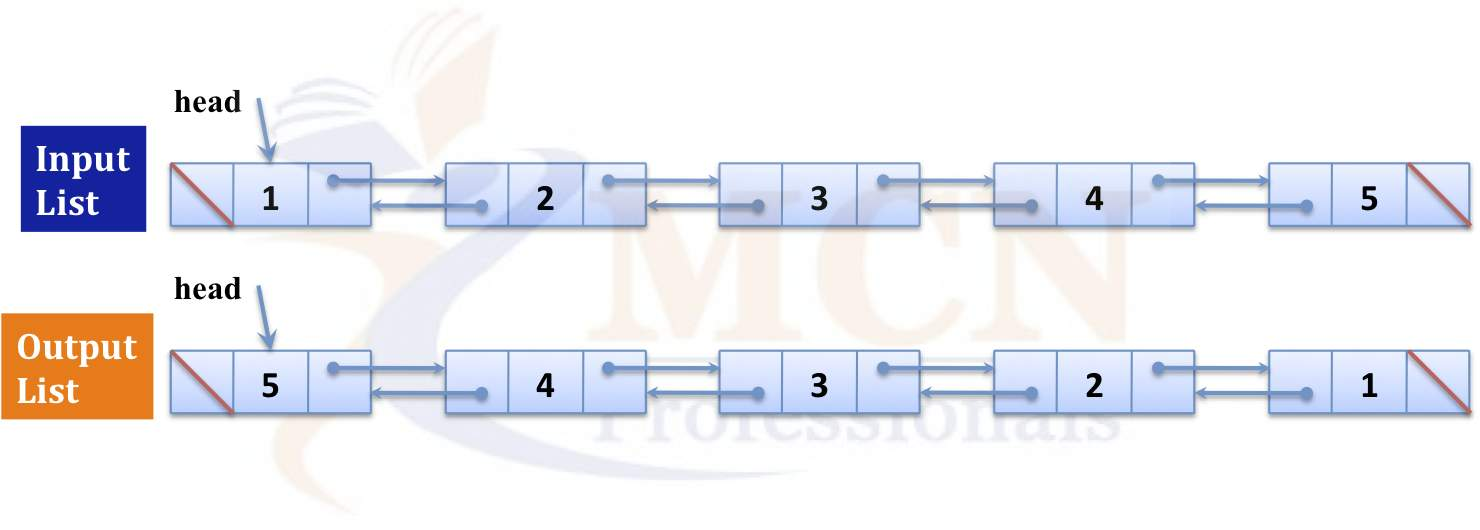# Reverse a Doubly Linked List

Problem Statement: Reverse a Doubly Linked List.

```Example:
Input: [1,2,3,4,5]
This means that you have given linked list of size 5  = 1 -> 2 -> 3 -> 4 -> 5 ;
Head is pointing towards 1 , and five is pointing to NULL ;

Output:   [5,4,3,2,1];
This means that after reversing the linked list should be like 5 -> 4 -> 3 -> 2 ->1 ;```

Disclaimer: Don’t jump directly to the solution, try it out yourself first.

## Approach:

We will use three-pointers to traverse through the entire list and interchange links between nodes of our Doubly Linked List

Step 1)

1. Pointer prev is pointing towards the NULL value of linked list as there is head .
2. Pointer curr is used to track the linked list .
3. And the pointer next is used to point to the next node which we have to reach .

Step 2)

1. Use a loop to iterate the Doubly linked list .
2. Then while iterating update the linked list pointer as followed
• Our temp will point towards current’s next node which is 2 is linked list
• Then current’s prev will point current ‘s next and current;s next will point temp
• Now current’s next will point toward temp ;(here we have changed the pointer of two nodes )
• Now remaining current will point prev;

Step 3)

1. Now checking a Edge condition what if our linked list is empty
2. So in that case we will check if our temp is pointing to NULL then we will reverse the pointer and point it towards prev ;

Code:

## C++ Code

``````#include<bits/stdc++.h>
using namespace std;
class node
{
public:
int data;
node *next;
node *prev;
node(int val)
{
data = val;
next = NULL;
prev = NULL;
}
};

// Reversing our double linked list
{
node*temp = NULL;

/* swap next and prev for all nodes of
doubly linked list */
while (curr != NULL)
{
temp = curr->prev;
curr->prev = curr->next;
curr->next = temp;
curr = curr->prev;
}

//Edge case if our linked list is empty Or list with only one node
if(temp != NULL )
}
// insertElement at end of our doubly linked list ;
void insertElement(node*&head, int val)
{
node * n = new node(val);
{
return ;
}
else {
while (temp->next != NULL)
{
temp = temp->next;
}
temp->next = n;
n->prev = temp;
}
}

// printing our double linked list
{
while (temp != NULL)
{
cout << temp->data << " ";
temp = temp->next;
}
cout << endl;
}
int main()
{
/* Start with the empty list */

cout << "Original Linked list" << endl;

/* Reverse doubly linked list */

cout << "Reversed Linked list" << endl;

return 0;
}
``````

Output:

1 2 3 4
4 3 2 1

Time Complexity: O(N) in Printing, reversing as well as insertion because we have to traverse all the nodes of linked list

Space Complexity: O(1)  we don’t use any extra space here;

## Java Code

``````import java.util.*;

class node
{
int data;
node next;
node prev;
node(int val)
{
data = val;
next = null;
prev = null;
}
}

class TUF{
// Reversing our double linked list
static node reverse(node head)
{
node temp = null;
node curr = head;

/* swap next and prev for all nodes of
doubly linked list */
while (curr != null)
{
temp = curr.prev;
curr.prev = curr.next;
curr.next = temp;
curr = curr.prev;
}

//Edge case if our linked list is empty Or list with only one node
if(temp != null )
}
// insertElement at end of our doubly linked list ;
static node insertElement(node head, int val)
{
node  n = new node(val);
{
}
else {
node temp = head;
while (temp.next != null)
{
temp = temp.next;
}
temp.next = n;
n.prev = temp;
}
}

// printing our double linked list
static void display(node head)
{
node temp = head;
while (temp != null)
{
System.out.print(temp.data+" ");
temp = temp.next;
}
System.out.println();
}
public static void main(String args[])
{
/* Start with the empty list */
node head = null;

/* Reverse doubly linked list */

}
}
``````

Output: